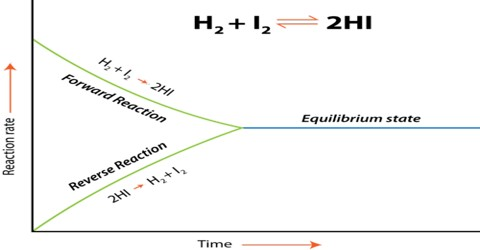Chemistry

# Activity and Activity Co-efficient in Chemical EquilibriumActivity and Activity Co-efficient in Chemical Equilibrium

It must be emphasized that the expressions for equilibrium constant

Equilibrium Law equations KC = {[L]le x [M]me} /{ [A]ae x [B]be}

and Gaseous Equilibria equation KP = [PlL x PmM] / [PaA x PbB]

are strictly valid for ideal systems, e.g., for ideal gases or gases at low pressures and ideal solutions. All real systems deviate from the ideal behavior, and for such systems activities (Section 15.13), instead of concentrations, are to be used in the expression for the equilibrium constant. The correct expression for the equilibrium constant then becomes,

K = [alL x amM] / [aaA x abB]

Here a, is the activity.

Activity of a constituent of a process may be looked upon as the effective concentration or idealized concentration which when replaced for the actual concentration in the expression for a physicochemical law will make the law apply exactly. When the pressure is high or the concentration is large the deviation from ideality increases and the activity differs considerably from concentration.

The ratio of the activity to the actual concentration is known as activity co-efficient, f. That is,

a/c = f

When f = 1, activity is equal to the concentration when a solution is very dilute and in the case of gases when the pressure is low.